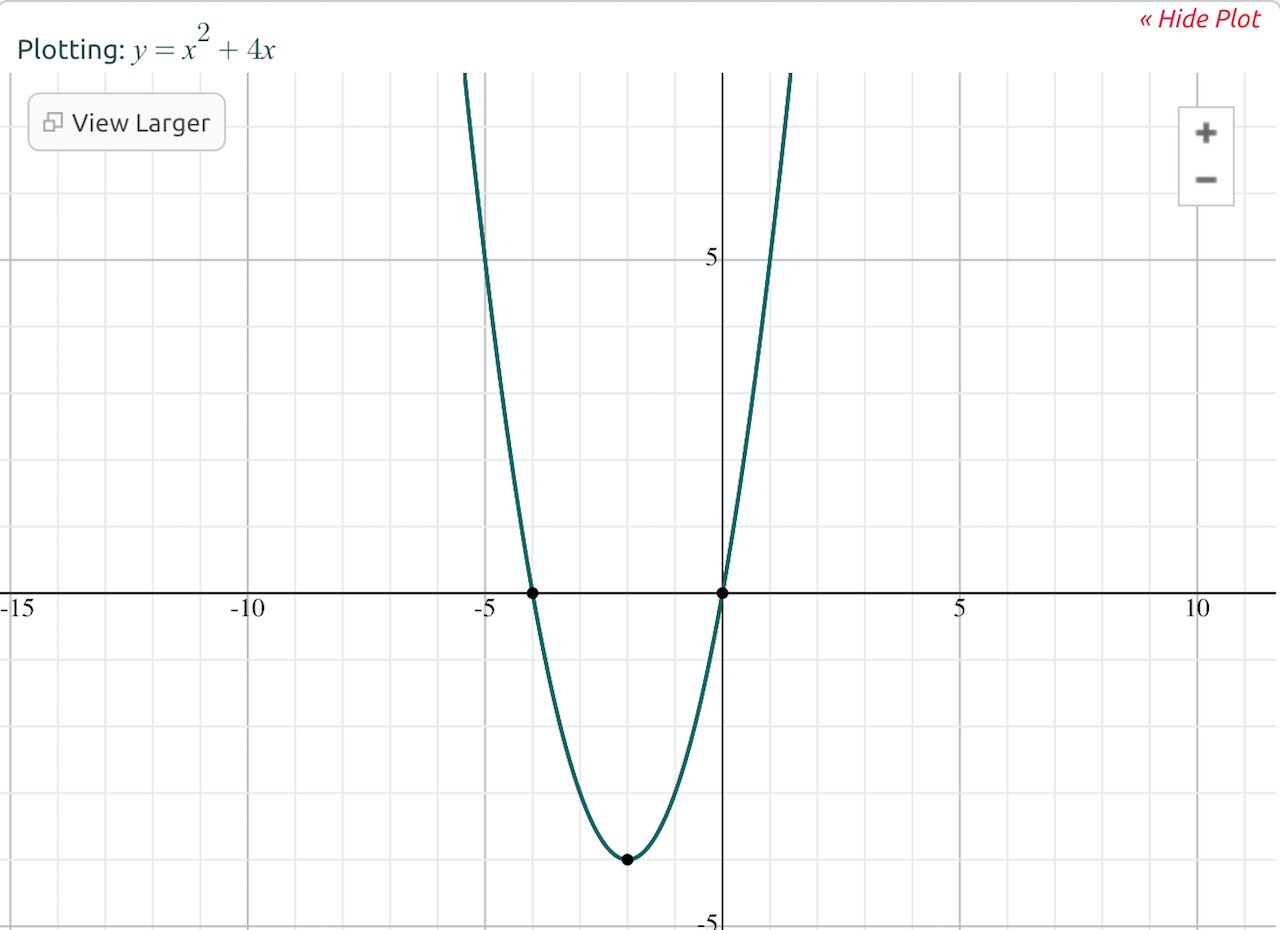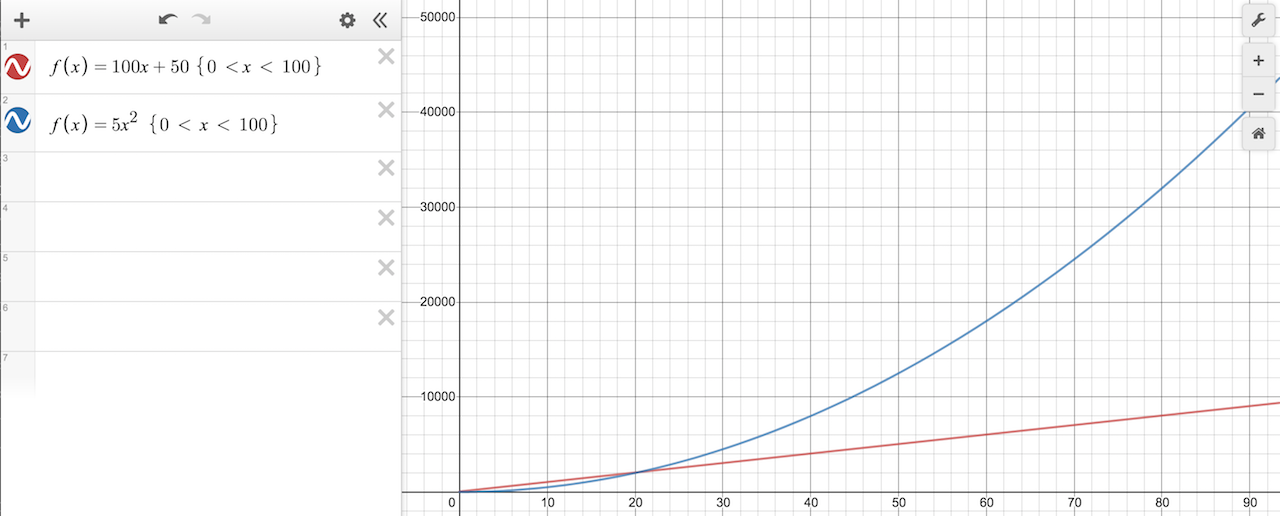# Exploring Big-O Notation With Ruby

You know Big-O is important - not only for acing your next job interview but for knowing how code works at scale. But have you ever taken the time to go beyond a superficial understanding of the subject? In this article, Julie Kent uses equal parts math and Ruby to reveal the beating heart of Big-O and show us how it ticks.

•By Julie Kent
• Feb 4, 2020

There once was a time when nothing scared me more than hearing the question, "What's the Big-O notation for that?" I remembered the topic from school, but because it had to do with math (which was never my strongest subject), I had blacked it out.

However, as my career progressed, I found myself:

• Looking at performance charts
• Trying to debug slow queries

When I decided it was time to circle back (get it?) to learn Big-O, I was surprised at its high-level simplicity. I'm sharing what I learned in this article so that you, my fellow engineers, can not only pass interviews with flying colors, but also build performant, scalable systems.

I promise, Big-O isn't as scary as it seems. Once you get it down, you can look at an algorithm and easily discern its efficiency, without having to run a profiling tool!

## What is Big-O notation?

Big-O notation is a fancy way to say, "Hey, what's the worst case performance for this algorithm?" You might have seen a function described as O(n) or O(1). This means:

• O(n) - Worst-case run time increases linearily as the input size (n) increases
• O(1) - Worst-case run time is constant for any size input

...and to really understand what this means, we need to learn about asymptotes

## Asymptotes?

Let's travel back to high school algebra, dust off our textbook and open it to the chapters on limits and asymptotes.

• Limit analysis: seeing what happens to a function as it approaches some value
• Asymptotic analysis: seeing what happens as f(x) approaches infinity

For example, let's say we plot the function f(x) = x^2 + 4x.We can perform the following analyses: - Limit analysis: As x increases, f(x) approaches infinity, so we can say that the limit of f(x) = x^2 + 4x as x approaches infinity is infinity. - Asymptotic analysis: As x becomes very large, the 4x term becomes insignificant compared to the x^2 term. So we can say that f(x) = x^2 + 4x becomes almost equivalent to f(x) = x^2 for values of x approaching infinity.

To understand how we can say that part of a function becomes "insignificant," consider what happens when you plug different numbers into the original function. For example, when x = 1, the function returns 1 + 4 (which equals 5). However, when x = 2,000, the function returns 4,000,000 + 8,000 (which equals 4,008,000) -- the x^2 term is contributing much more to the total than the 4x.

Big-O notation is one way of describing how an algorithm's run time changes as the size of the input changes.

## What determines the run time of an algorithm?

If I ask you how long it will take you to find a needle in a haystack, I imagine you will want to know how much hay is in the stack. If I respond "10 pieces" I bet you will be pretty confident you can find the needle within a minute or two, but if I say "1,000 pieces," you probably won't be as excited.

There's one other piece of information you should know. How much longer does it take to search the stack for each piece of hay added? And what happens as the amount of hay approaches infinity?

This is very similar to the example of asymptotic analysis above. Let's look at one more example just to make sure we've all got this down. Consider the function f(x) = 5x^2 + 100x + 50. We can plot the two parts of this function separately:Just like the previous example, the 5x^2 term eventually becomes larger than the 100x + 50 terms, so we can drop them and say that the runtime of f(x) = 5x^2 + 100x + 50 grows as x^2.

Of course, it's worth mentioning that there are other factors affecting run time, such as the speed of the actual computer running the program, and the language used.

Let's do a Big-O analysis of the linear search algorithm. A linear search starts at the beginning of a dataset and traverses until the target element is found.

Here is an implementation in Ruby.

``````def find_number_via_linear_search(array, target)
counter = 0

# iterate through the given array starting
# at index 0 and continuing until the end
while counter < array.length
if array[counter] == target
# exit if target element found
return "linear search took: #{counter} iterations"
else
counter += 1
end
end

end
``````

We could give this method a spin like this:

``````# Let's create an array with 50 integers
# and then re-arrange the order to make
# things more interesting
array = [*1..50].shuffle

find_number_via_linear_search(array, 24)
``````

I ran this a few times and got the following results:

``````=> "linear search took: 10 iterations"
=> "linear search took: 11 iterations"
=> "linear search took: 26 iterations"
``````

When analyzing the Big-O notation of a function, we care about the worst-case scenerio (a.k.a. the upper asymptotic bound).

Thinking about this intuitively, the smallest number of iterations would be 1. That occurrs when the target element was in position 0 in the array. The largest number of iterations (or worst-case scenerio) would be 50. This will happen when the target element was not found in the array.

If our array has 100 elements, the worst-case will be 100 iterations. 200 elements? 200 iterations. Thus, the Big-O notation for linear search is simply O(n), where n is the number of elements.

Let's consider binary search next. Here's how you do a binary search of a pre-sorted array: 1. Take the middle element
2. If `element == target` we're done 3. If `element > target` discard the top half of the array 4. If `element < target` discard the bottom half of the array 5. Start over at step 1 with the remaining array

Note: If you're a Rubyist, there is a built-in b-search method that implements this algorithm for you!

For example, let's say that you have a dictionary and you're looking for the world "pineapple." We'd go to the middle page of the dictionary. If it happened to have the world "pineapple," we'd be done!

But my guess is, the middle of the dictionary wouldn't be in the "p's" yet so maybe we'd find the word "llama." The letter "L" comes before "P" so we would discard the entire lower half of the dictionary. Next, we'd repeat the process with what remained.

As with linear search, the best-case run time for a binary search is one iteration. But what's the worst case? Here is an example array that has 16 elements -- let's pretend that we want to use a binary search to find the number 23:

``````[2, 3, 15, 18, 22, 23, 24, 50, 65, 66, 88, 90, 92, 95, 100, 200]
``````

The first step would be to look at the number at index 7, which is 50. Since 50 is greater than 23, we discard everything to the right. Now our array looks like this:

``````[2, 3, 15, 18, 22, 23, 24, 50]
``````

The middle element is now 18, which is less than 23, so we discard the lower half this time.

``````[22, 23, 24, 50]
``````

Which becomes

``````[22, 23]
``````

Which finally becomes

``````
``````

In total, we had to divide the array in half 4 times in order to find the number we were targeting in the array, which had a length of 16.

To generalize this, we can say that the worst case scenerio for binary search is equal to the maximum number of times we can divide the array in half.

In math, we use logarithms to answer the question, "How many of this number do we multiply to get that number?" Here's how we apply logarithms to our problem:We can thus say that the Big-O, or worst-case run time for a binary search, is log(base 2) n.

## Wrapping up

Big-O notation is a fancy way to say, "Hey, what's the worst case for this?" Putting computer science aside, a real-world example could be what happens when you ask your plumber how much it will cost to repair the broken faucet. He might respond, "Well, I can guarantee it won't be more than \$2,000." This is an upper bound, although not very helpful to you.

Because of this, other Big-O notations are often utilized. For example, Big-Theta cares about both the lower bound and upper bound. In this case, the plumber would respond, "Well, it won't be less than \$1,000 but it won't be more than \$2,000." This is much more useful.

Thank you for reading, and I hope that this post helped make Big-O notation at least a slightly less scary topic!

What to do next:
Honeybadger helps you find and fix errors before your users can even report them. Get set up in minutes and check monitoring off your to-do list.
Easy 5-minute setup — No credit card required
Each month we share news, best practices, and stories from the DevOps & monitoring community—exclusively for developers like you.#### Julie Kent

Julie is an engineer at Stitch Fix. In her free time, she likes reading, cooking, and walking her dog.

More articles by Julie Kent
Stop wasting time manually checking logs for errors!

Try the only application health monitoring tool that allows you to track application errors, uptime, and cron jobs in one simple platform.

• Know when critical errors occur, and which customers are affected.
• Respond instantly when your systems go down.
• Improve the health of your systems over time.
• Fix problems before your customers can report them!

As developers ourselves, we hated wasting time tracking down errors—so we built the system we always wanted.

Honeybadger tracks everything you need and nothing you don't, creating one simple solution to keep your application running and error free so you can do what you do best—release new code. Try it free and see for yourself.

Simple 5-minute setup — No credit card requiredMolly Struve, Sr. Site Reliability Engineer, Netflix